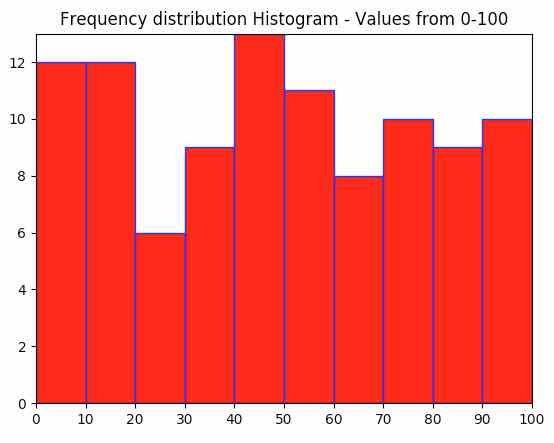# Histogram Plotting With Matplotlib

## Histogram Overview:

• A Histogram is a vertical bar chart.
• A Histogram has continuous intervals in the X-axis and the frequencies in the Y-axis.
• While X-axis of the histogram describes the interval of the data that is start value and end value of the data, the Y axis of a histogram describes the frequencies of such data.
• In other words the height of the histogram describes the frequency of the data and the width of a rectangle in the histogram describes the range of values of data.
• Histogram gives a good overview of how the density of the data is distributed.
• The python library matplotlib has the hist() function and a hist2d() function that plot a histogram.

## Example:

 import matplotlib.pyplot as plot import numpy as np   # Random number generation from 0 to 100 x1      = np.random.random_integers(0,100,100)   # Plot frequency distribution using histogram plot.hist(x1, facecolor="r", edgecolor='b') plot.title("Frequency distribution Histogram - Values from 0-100") plot.margins(0) plot.xticks(range(0,110,10))   # Display the histogram plot.show()

## Output: